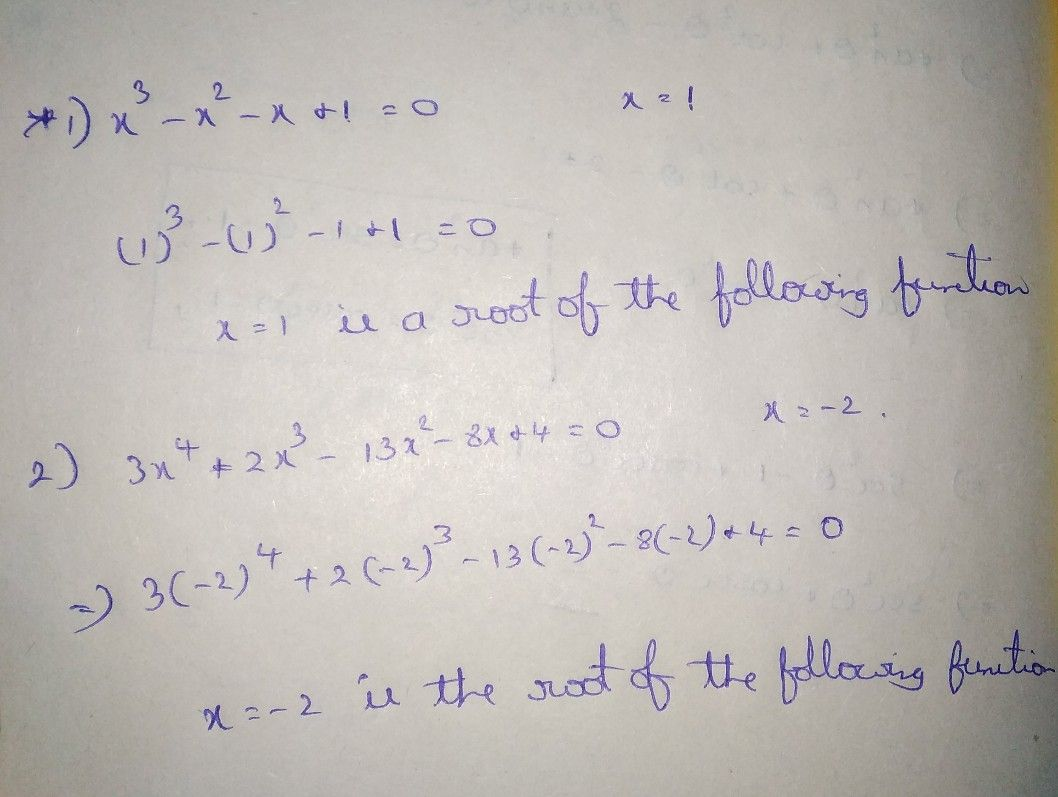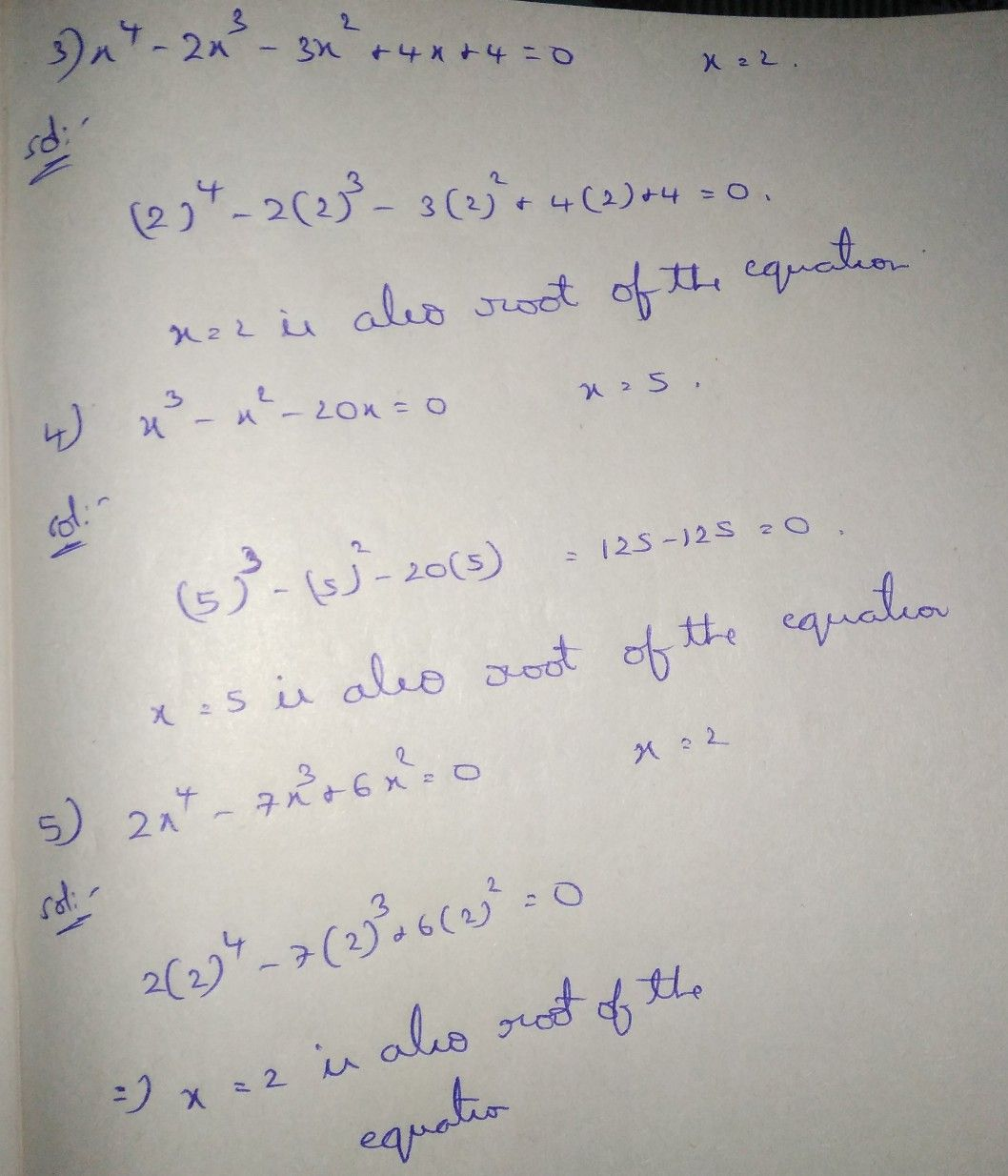Symbol
ProblemLearning Task $2.$ Find the roots of the following polynomial equations given one of its roots $on$ the right side. $1$ $x^{3}-x^{2}-x+1=0$ $x=1$ $2$ $3x^{4}+2x^{3}-13x^{2}-8x+4=0$ $x=-2$ $3$ $x^{4}-2x^{3}-3x^{2}+4x+4=0$ $x=2$ $4$ $x^{3}-x^{2}-20x=0$ $x=5$ $5$ $2x^{4}-7x^{3}+6x^{2}=0$ $x=2$
10th-13th grade
Other
Search count: 122
Question content
Find the roots of the given polynomial equations given one of its roots on the right side.
SolutionQanda teacher - AravindStudent
thanks ?✨